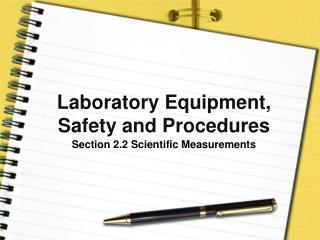Download PresentationLaboratory Equipment, Safety and ProceduresLaboratory Equipment, Safety and Procedures - PowerPoint PPT Presentation

Download PresentationLaboratory Equipment, Safety and Procedures
An Image/Link below is provided (as is) to download presentation

Download Policy: Content on the Website is provided to you AS IS for your information and personal use and may not be sold / licensed / shared on other websites without getting consent from its author. While downloading, if for some reason you are not able to download a presentation, the publisher may have deleted the file from their server.

- - - - - - - - - - - - - - - - - - - - - - - - - - - E N D - - - - - - - - - - - - - - - - - - - - - - - - - - -
Presentation Transcript

1. Laboratory Equipment, Safety and Procedures Section 2.2 Scientific Measurements

2. Pre-View 2.2 • Mass – the measure of how much matter is in an object • Gram – SI unit for mass • Scale balance – used to measure mass • Triple beam balance – a type of scale balance commonly used in high school laboratories • Weight – the measurement of force exerted by gravity on an object

3. Pre-View 2.2 • Triple beam balance – a type of scale balance commonly used in high school laboratories • Newton – SI unit for force (and weight) • Spring scale – equipment used to find force and weight • Ruler or metric stick – equipment used in the laboratory to measure length in millimeters, centimeters, or meters

4. Scientific Measurements • Weight and mass are not the same – what is the difference between the two? • Mass is the measurement of how much matter is in an object. • It is measured in grams using a scale balance such as a triple beam balance.

5. Scientific Measurements • Weight is a measurement of the force of gravity on an object • It is measured in newtons using a spring scale. • If you went to the moon where the gravity is only about 20% of the earth’s gravity, your mass would not change since your body would contain the same amount of matter, but your weight would be less on the moon than on eart h due to gravity.

6. Equipment for Measuring Mass • A scale balance is used to find the mass of an object. • Most common types of scale balances are the triple beam balance and the electronic balance. • Both measure mass in grams.

7. Triple Beam Balance

8. Using a Triple Beam Balance Step 1 • Be sure the balance is on a level surface. • Move the three sliders as far left as they will go prior to placing anything on the pan. • Indicator on the right should be on the zero mark. • If not, calibrate the balance by turning the screw under the pan until it is in line.

9. Using a Triple Beam Balance Step 2 • Place the object being measured on the pan and move the 100 gram slider on the beam until the indicator drops below the mark. • Be sure it clicks into place. • The number to the left of this point will show the number of hundreds of grams in the object. • Move the slider back one notch to the left so that the indicator is once again above or equal to the zero mark. • The slider should now point to the number of hundreds of grams in the object.

10. Using a Triple Beam Balance Step 3 • Move the 10 gram slider along its beam until the indicator drops below zero. • Be sure the slider clicks into place. • Once again the number to the left of this point will tell you how many tens of grams are in the object. • Move the slider back one notch to the left so that the indicator is above or equal to the zero mark. • This slider will now point to the number of tens of grams in the object.

11. Using a Triple Beam Balance Step 4 • The one gram slider is not notched, so you can move it anywhere on the beam. • The numbers marked on this beam are grams, and the marks between are tenths of a gram. • Move this slider until the indicator exactly lines up with the zero mark. • The object’s mass now “balances” the mass on the beams. • By adding the numbers together, you can find the mass of the object.

12. Equipment for Measuring Weight or Force • Weight is a measurement of the force of gravity on an object, and it is measured in newtons (the SI unit for force). • Weight and force are measured using a spring scale. • Your bathroom scale is a spring scale. • Some scales have a dial readout, and others have a linear scale.

13. Using a Spring Scale • To find the weight of an object using this spring scale, you would hold the scale up and attach the object to be weighed to the hook at the bottom. • The spring will stretch, and the pointer will move along the scale and point to the number that shows the object’s weight.